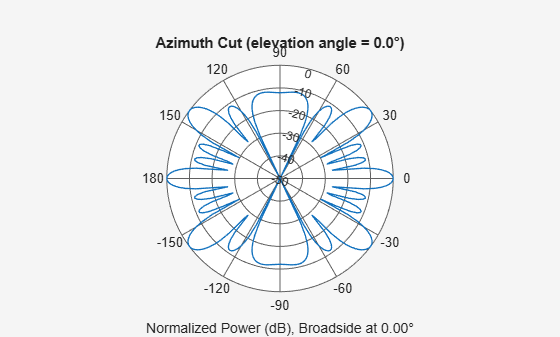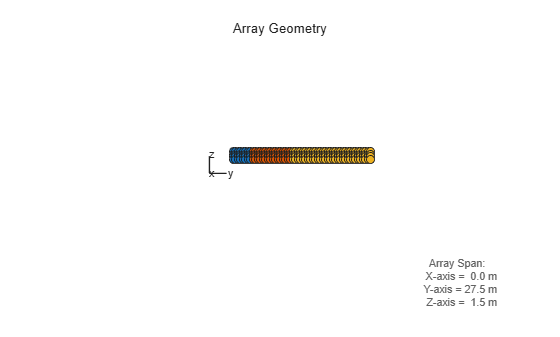Main Content

# phased.PartitionedArray

Phased array partitioned into subarrays

## Description

The `PartitionedArray` object represents a phased array that is partitioned into one or more subarrays.

To obtain the response of the subarrays in a partitioned array:

1. Define and set up your partitioned array. See Construction.

2. Call `step` to compute the response of the subarrays according to the properties of `phased.PartitionedArray`. The behavior of `step` is specific to each object in the toolbox.

You can also specify a `PartitionedArray` object as the value of the `SensorArray` or `Sensor` property of objects that perform beamforming, steering, and other operations.

Note

Starting in R2016b, instead of using the `step` method to perform the operation defined by the System object™, you can call the object with arguments, as if it were a function. For example, `y = step(obj,x)` and `y = obj(x)` perform equivalent operations.

## Construction

`H = phased.PartitionedArray` creates a partitioned array System object, `H`. This object represents an array that is partitioned into subarrays.

`H = phased.PartitionedArray(Name,Value)` creates a partitioned array object, `H`, with each specified property Name set to the specified Value. You can specify additional name-value pair arguments in any order as (`Name1`,`Value1`,...,`NameN`,`ValueN`).

## Properties

 `Array` Sensor array Sensor array, specified as any array System object belonging to Phased Array System Toolbox™. Default: `phased.ULA('NumElements',4)` `SubarraySelection` Subarray definition matrix Specify the subarray selection as an M-by-N matrix. M is the number of subarrays and N is the number of elements in the array. Each row of the matrix corresponds to a subarray and each entry in the row indicates whether or not an element belongs to the subarray. When the entry is zero, the element does not belong to the subarray. A nonzero entry represents a complex-valued weight applied to the corresponding element. Each row must contain at least one nonzero entry. The phase center of each subarray is at the subarray geometric center. The `SubarraySelection` and `Array` properties determine the geometric center. Default: `[1 1 0 0; 0 0 1 1]` `SubarraySteering` Subarray steering method Specify the method of subarray steering as either `'None'` | `'Phase'` | `'Time'` | `'Custom'`. When you set this property to `'Phase'`, a phase shifter is used to steer the subarray. Use the `STEERANG` argument of the `step` method to define the steering direction.When you set this property to `'Time'`, subarrays are steered using time delays. Use the `STEERANG` argument of the `step` method to define the steering direction.When you set this property to `'Custom'`, subarrays are steered by setting independent weights for all elements in each subarray. Use the `WS` argument of the `step` method to define the weights for all subarrays. Default: `'None'` `PhaseShifterFrequency` Subarray phase shifter frequency Specify the operating frequency of phase shifters that perform subarray steering. The property value is a positive scalar in hertz. This property applies when you set the `SubarraySteering` property to `'Phase'`. Default: `300e6` `NumPhaseShifterBits` Number of phase shifter quantization bits The number of bits used to quantize the phase shift component of beamformer or steering vector weights. Specify the number of bits as a non-negative integer. A value of zero indicates that no quantization is performed. Default: `0`

## Methods

Specific to `phased.PartitionedArray` Object
`beamwidth`

Compute and display beamwidth for a subarray

`collectPlaneWave`

Simulate received plane waves

`directivity`

Directivity of partitioned array

`getElementPosition`

Positions of array elements

`getNumElements`

Number of elements in array

`getNumSubarrays`

Number of subarrays in array

`getSubarrayPosition`

Positions of subarrays in array

`isPolarizationCapable`

Polarization capability

`pattern`

Plot partitioned array directivity, field, and power patterns

`patternAzimuth`

Plot partitioned array directivity or pattern versus azimuth

`patternElevation`

Plot partitioned array directivity or pattern versus elevation

`plotResponse`

Plot response pattern of array

`step`

Output responses of subarrays

`viewArray`

View array geometry

Common to All System Objects
`release`

Allow System object property value changes

## Examples

collapse all

Plot the azimuth response of a 4-element ULA partitioned into two 2-element ULA's. The element spacing is one-half wavelength.

Create the ULA, and partition it into two 2-element ULA's.

```sULA = phased.ULA('NumElements',4,'ElementSpacing',0.5); sPA = phased.PartitionedArray('Array',sULA,... 'SubarraySelection',[1 1 0 0;0 0 1 1]);```

Plot the azimuth response of the array. Assume the operating frequency is 1 GHz and the propagation speed is the speed of light.

```fc = 1e9; pattern(sPA,fc,[-180:180],0,'Type','powerdb',... 'CoordinateSystem','polar',... 'Normalize',true)```Create a 4-element ULA. Then partition the ULA into two 2-element ULAs. Then, calculate the response at boresight of a 4-element ULA partitioned into two 2-element ULAs.

```sULA = phased.ULA('NumElements',4,'ElementSpacing',0.5); sPA = phased.PartitionedArray('Array',sULA,... 'SubarraySelection',[1 1 0 0;0 0 1 1]);```

Calculate the response at 1 GHz. The signal propagation speed is the speed of light.

```fc = 1e9; resp = step(sPA,fc,[0;0],physconst('LightSpeed'))```
```resp = 2×1 2 2 ```

Create a partitioned URA array with three subarrays of different sizes. The subarrays have 8, 16, and 32 elements. Use different sets of subarray element weights for each subarray.

Create a 4-by-56 element URA.

```antenna = phased.IsotropicAntennaElement; fc = 300e6; c = physconst('LightSpeed'); lambda = c/fc; n1 = 2^3; n2 = 2^4; n3 = 2^5; nrows = 4; ncols = n1 + n2 + n3; array = phased.URA('Element',antenna,'Size',[nrows,ncols]);```

Select the three subarrays by setting the selection matrix.

```sel1 = zeros(nrows,ncols); sel2 = sel1; sel3 = sel1; sel = zeros(3,nrows*ncols); for r = 1:nrows sel1(r,1:n1) = 1; sel2(r,(n1+1):(n1+n2)) = 1; sel3(r,((n1+n2)+1):ncols) = 1; end sel(1,:) = sel1(:); sel(2,:) = sel2(:); sel(3,:) = sel3(:);```

Create the partitioned array.

```partarray = phased.PartitionedArray('Array',array, ... 'SubarraySelection',sel,'SubarraySteering','Custom'); viewArray(partarray,'ShowSubarray','All');```Set weights for each subarray and get the response of each subarray. Put the weights in a cell array.

```wts1 = ones(nrows*n1,1); wts2 = 1.5*ones(nrows*n2,1); wts3 = 3*ones(nrows*n3,1); resp = partarray(fc,[30;0],c,{wts1,wts2,wts3})```
```resp = 3×1 complex 0.0246 + 0.0000i 0.0738 - 0.0000i 0.2951 - 0.0000i ```

 Van Trees, H.L. Optimum Array Processing. New York: Wiley-Interscience, 2002.

## Support

#### Exploring Hybrid Beamforming Architectures for 5G Systems

Download white paper